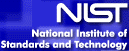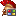# Case study: Load Cell CalibrationNIST info: This example illustrates the construction of a linear regression model for load cell data that relates a known load applied to a load cell to the deflection of the cell. The model is then used to calibrate future cell readings associated with loads of unknown magnitude. Full description can be found here.

Data: The data collected in the calibration experiment consisted of a known load, applied to the load cell, and the corresponding deflection of the cell from its nominal position. Forty measurements were made over a range of loads from 150,000 to 3,000,000 units. The data were collected in two sets in order of increasing load. The systematic run order makes it difficult to determine whether or not there was any drift in the load cell or measuring equipment over time. Assuming there is no drift, however, the experiment should provide a good description of the relationship between the load applied to the cell and its response. Full dataset is available here.

In this example we will follow the suggested steps, listed at NIST pages. As suggested, we will use linear and quadratic polynomial to perform fitting and estimating.

1) Read in the data: For regression we will use Polynoms PolyFit routine. First we have to create necessary vectors and populate them.

2) Plot the y=y(x) chart to get an idea about the fit model. For this, you can use the MtxVecTee.DrawValues method. These two steps can be acomplished by the following code:``````Uses MtxVec, Polynoms, Math387, MtxVecTee, MtxExpr;

procedure FitModel;
var  x,y, residuals, coeffs, ycalc: Vector;
begin
nlr := TMtxNonLinReg.Create(nil);
Chart1.FreeAllSeries;
try
DrawValues(x,y,Chart1.Series);````````````#include "MtxVecCpp.h"
#include "Polynoms.hpp"
#include "Math387.hpp"
#include "MtxVec.hpp"
#include "MtxVecTee.hpp"

void FitModel(void);
{
Vector x,y,residuals,coeffs,ycalc;
Chart1->FreeAllSeries();
try
{
DrawValues(x,y,Chart1->Series,0,1,false);````````````using Dew.Math;
using Dew.Math.Units;
using Dew.Stats.Units;
using Dew.Stats;

namespace Dew.Tests
{
private void FitModel()
{
Vector x = new Vector(0);
Vector y = new Vector(0);
Vector residuals = new Vector(0);
Vector ycalc = new Vector(0);
Vector coeffs = new Vector(0);
tChart1.Series.Clear();
try
{
MtxVecTee.DrawValues(x, y, tChart1,0,1,false);
``````

Plotting the data indicates that the hypothesized, simple relationship between load and deflection is reasonable. The plot indicates that a straight-line model is likely to fit the data. It does not indicate any other problems, such as presence of outliers or nonconstant standard deviation of the response.

3) Perform simple LQ linear regression on data: With just few lines of code, least squared linear regression is applied to x,y data:``````
// Perform linear regression
PolyFit(x,y,coeffs,1);
// Calculate residuals
PolyEval(x,coeffs,ycalc);
residuals.Sub(ycalc,y);``````
// perform linear regression
PolyFit(x,y,coeffs,1);
// calculate residuals
PolyEval(x,coeffs,ycalc);
residuals->Sub(ycalc,y);``````// Perform linear regression
Polynoms.PolyFit(x,y,coeffs,2);
// Calculate residuals
Polynoms.PolyEval(x,coeffs,ycalc);
residuals.Sub(ycalc,y);